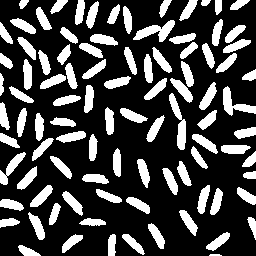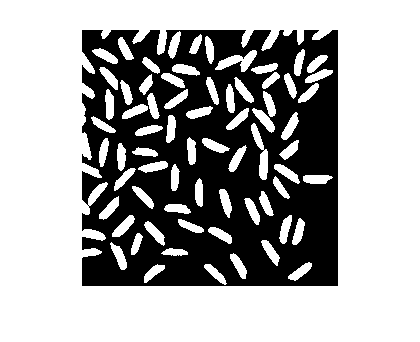# Counting objects without bias

In today's post I want to look at two methods for counting objects per unit area. For example, how can we estimate the number of objects per unit area in this image:I could simply count the number of connected components and divide the total image area. (For this post I'll assume that each pixel has an area of 1.0.)

url = 'https://blogs.mathworks.com/images/steve/2012/rice-bw-2.png';
cc = bwconncomp(bw)

cc =

Connectivity: 8
ImageSize: [256 256]
NumObjects: 95
PixelIdxList: {1x95 cell}


objects_per_unit_area = cc.NumObjects / (size(bw,1) * size(bw,2))

objects_per_unit_area =

0.0014



This method, however, is wrong according to The Image Processing Handbook, John C. Russ, 5th ed., CRC Press, 2007, pp. 565-567. "When features intersect the edge of the field of view, it is not proper to count all features that can be seen." Russ gives two better methods.

The first method ignores objects touching a pair of adjacent edges, such as the top and left edges. Russ points out that this is "equivalent to counting each feature by its lower right corner," and he draws an analogy to counting people in a room by counting noses. (I'll have to ask the developers on the Computer Vision System Toolbox team for help with nose detection.)

The Image Processing Toolbox has a function, imclearborder, for removing all objects touching the image borders. With a little creativity, we can use that function to identify all objects that do not touch the top and left borders.

We start by padding the image with 0s on the left and top.

bw2 = padarray(bw,[1 1],0,'pre');


Because of the padding, there are no objects touching the left and top borders of bw2. Calling imclearborder on this image, then, will remove all objects touching the bottom and right borders in the original image, but it will leave objects touching the top and left borders alone.

bw3 = imclearborder(bw2);


Now let's remove the padded column on the left and padded row on the top.

bw4 = bw3(2:end,2:end);
imshow(bw4)What's the object count per unit area now?

cc = bwconncomp(bw4);
objects_per_unit_area_2 = cc.NumObjects / (size(bw4,1) * size(bw4,2))

objects_per_unit_area_2 =

0.0013



The first method, which counted all objects in the original image, was off by somewhere in the neighborhood of 10-11%.

abs(objects_per_unit_area_2 - objects_per_unit_area) / ...
objects_per_unit_area

ans =

0.1053



Another method described by Russ is to do a weighted count of all objects that do not touch any edge. The weight count compensates for the relative likelihood that an object with a certain horizontal and vertical extent would touch the edge of a certain field of view. The equation for the weighted count of each object is

$$C = \frac{W_x W_y}{(W_x - F_x) (W_y - F_y)}$$

where $W_x$ and $W_y$ are the dimensions of the image in the x and y directions, and $F_x$ and $F_y$ are the maximum projected dimensions of the object in those directions.

We make use of the Image Processing Toolbox function regionprops to compute the bounding box of each object, from which we can determine $F_x$ and $F_y$.

Start by clearing all objects that touch any image border.

bw5 = imclearborder(bw);


Next, compute the bounding boxes of all the remaining objects.

s = regionprops(bw5,'BoundingBox');


For example, here's the bounding box of the fifth detected object:

s(5)

ans =

BoundingBox: [17.5000 166.5000 20 24]



I'm going to use an advanced bit of MATLAB syntax here to convert the structure array s into an ordinary P-by-4 matrix containing all the bounding boxes together. The second line of code displays the bounding boxes of the first seven objects.

bboxes = cat(1,s.BoundingBox);
bboxes(1:7,:)

ans =

9.5000   86.5000   25.0000   17.0000
10.5000   18.5000   26.0000   16.0000
17.5000   37.5000   19.0000   23.0000
17.5000  108.5000   10.0000   29.0000
17.5000  166.5000   20.0000   24.0000
23.5000   59.5000    9.0000   31.0000
28.5000  212.5000   17.0000   28.0000



$F_x$ is the third column, and $F_y$ is the fourth column.

Fx = bboxes(:,3);
Fy = bboxes(:,4);


$W_x$ and $W_y$ are computed from the size of the image.

[Wy,Wx] = size(bw);


Now compute a vector of weighted counts, one for each object that doesn't touch a border.

C = (Wx * Wy) ./ ((Wx - Fx) .* (Wy - Fy));
C(1:7)

ans =

1.1871
1.1872
1.1868
1.1736
1.1970
1.1792
1.2027



The last step is to sum the counts and divide by the total image area.

objects_per_unit_area_3 = sum(C) / (Wx*Wy)

objects_per_unit_area_3 =

0.0012



Russ comments that this last method may work better for images containing nonconvex objects that may touch image boundaries more than once.